# Solve Equations by Multiplying and Dividing

//Solve Equations by Multiplying and Dividing
Solve Equations by Multiplying and Dividing 2017-11-13T22:01:52+00:00

Algebra tiles can help you visualize what’s actually happening when you are solving algebraic equations.

Tips: Be sure the tile itself and not the text is selected when making a copy.

You can:

• Right click and copy
• Select the tile and press Ctrl + D
• Use the Ctrl + C, Ctrl + V copy and paste short cuts or
• Hold the Ctrl key down while dragging a tile which also makes a copy of the original

The Division Property of Equality states

For all real numbers a, b, and c, if a = b, then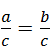Solve 3x = 15 using Algebra Tiles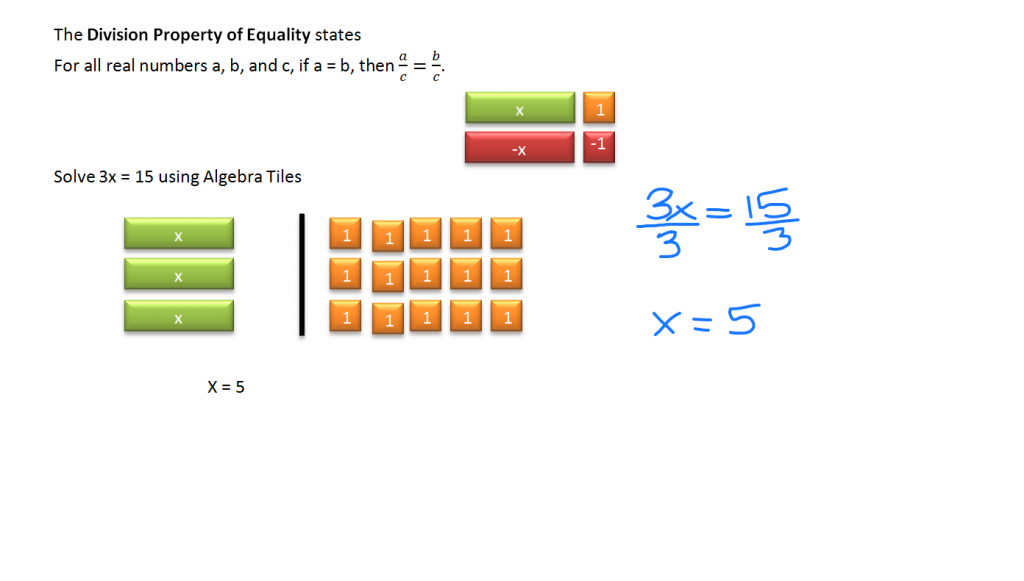The black bar stands for the equal sign. The first step is to copy the tiles above to model the equation. Once the equation has been formed, the next step is to isolate the variable x. There are three x tiles equal to 15 ones tiles. Place the ones tiles in such a way that they are evenly split among the x tiles. Since each x tile corresponds to five ones tiles, x = 5.

Solve 2x = -3.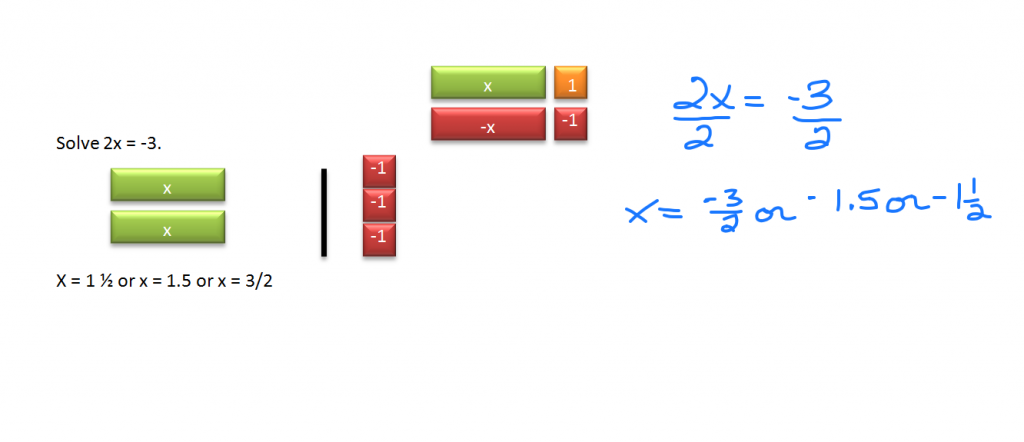Start by gathering the tiles needed. Place two positive x tiles on the left and three ones tiles on the right. Line the ones tiles up vertically since they won’t split up evenly. We have more than one ones tile for each x tile but less than two. The value of x is 1 and a half tiles so x = 1 ½ or x = 1.5, or you can write x = 3/2. All are equivalent and acceptable answers.

Solve -1 = 2x + 5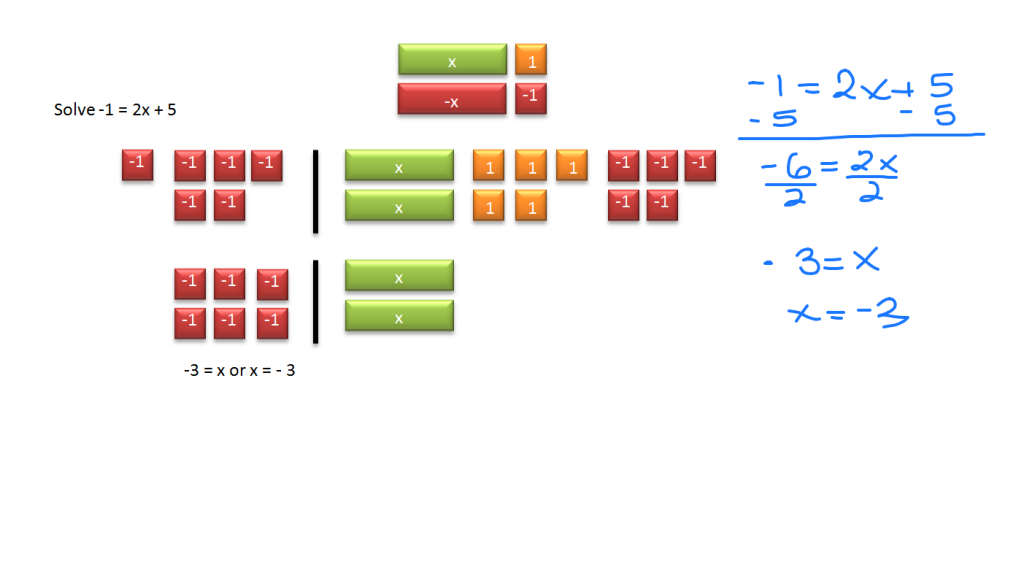In this example, there is an extra step needed. Copy the needed tiles on each side of the equals sign. Not only do we have two x tiles to contend with, but we have five ones tiles added to the two x tiles. Start by isolating the 2x. If five is added to it, how do you eliminate them? Put five negative one tiles on the right. Remember that with the Addition Property of Equality, whatever you do to one side of the equation must be done to the other. Put five negative one tiles on the left side as well. The zero pairs cancel each other out. We are left with six negative one tiles on the left and two positive x tiles on the right. Line them up so they are equally divided between the x tiles. Since -6 = 2x, each one x equals three negative one tiles. So -3 = x or x = -3 if you prefer to write it that way.

Solve x/3 = -2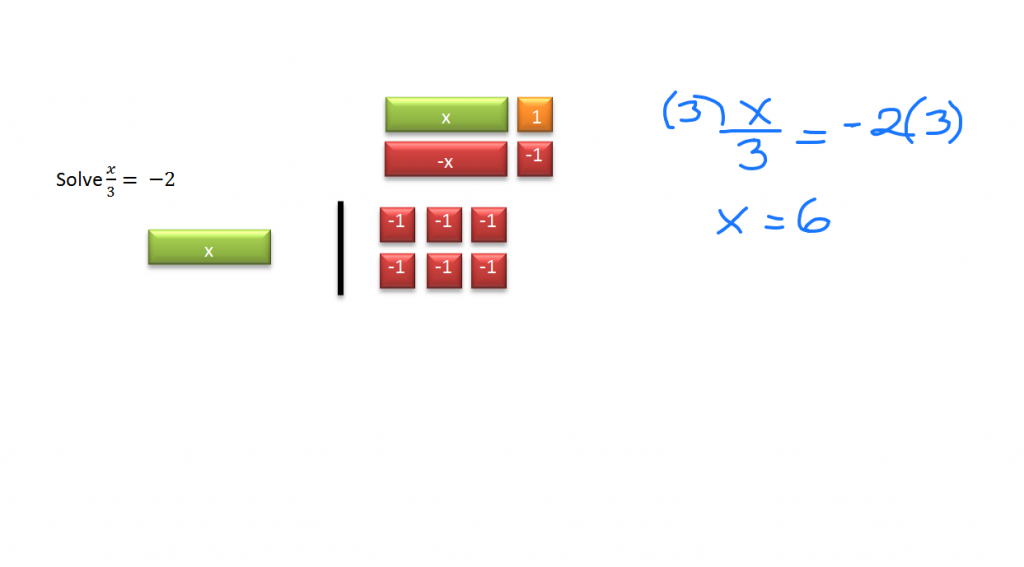Division is a bit harder to model using tiles. The x tile is split evenly into three parts. The two negative one tiles are on the right side of the equals sign. To get a single x tile on the left, we need three times as many ones tiles on the right. So a single x tile is equivalent to six negative one tiles.

Solving Equations via Multiplication and Division Demo

Holt, Rinehart and Winston Algebra 1 p 122 – 128